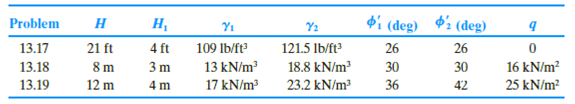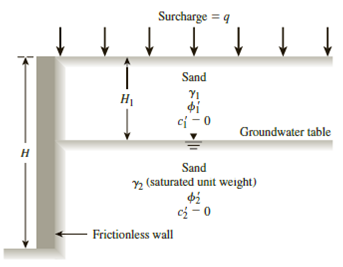Chapter 13, Problem 13.17PPrinciples of Geotechnical Enginee...

9th Edition
Braja M. Das + 1 other
ISBN: 9781305970939

Solutions

Chapter
SectionPrinciples of Geotechnical Enginee...

9th Edition
Braja M. Das + 1 other
ISBN: 9781305970939
Textbook Problem

13.17 through 13.19 A retaining wall is shown in Figure 13.41. For each problem, determine the Rankine active force, Pa, per unit length of the wall and the location of the resultant.Figure 13.41

To determine

Find the Rankine active force Pa per unit length of the wall and the location z¯ of the resultant force.

Explanation

Given information:

The height (H) of the retaining wall is 21 ft.

The depth H1 of sand is 4.0 ft.

The unit weight γ1 of the sand is 109lb/ft3.

The sand friction angle ϕ1 is 26°.

The saturated sand friction angle ϕ2 is 26°.

The cohesion c1 of sand is 0.

The surcharge pressure (q) is 0.

The depth H2 of saturated sand is 4.0 ft.

The saturated unit weight γ2 of the sand is 121.5lb/ft3.

The cohesion c2 of saturated sand is 0.

Calculation:

Determine the active earth pressure coefficient Ka using the formula.

Ka=tan2(45ϕ2)

Substitute 26° for ϕ.

Ka=tan2(4526°2)=tan2(4513)=tan2(32)=0.39

Determine the total stress σo at 0 ft depth using the relation.

σo=0

Determine the pore water pressure at 0 ft depth using the relation.

u=γw×h

Here, γw is the unit weight of the water.

Take the unit weight of the water as 62.4lb/ft3.

Substitute 62.4lb/ft3 for γw and 0 ft for h.

u=62.4×0=0

Determine the effective active earth pressure σa at 0 ft depth using the relation.

σa=σoKa

Substitute 0 for σo and 0.39 for Ka.

σa=0(0.39)=0

Determine the total stress σo at 4 ft depth using the relation.

σo=γ1×H1

Substitute 109lb/ft3 γ1 and 4.0 ft for H1.

σo=109×4.0=436lb/ft2

Determine the pore water pressure at 4 ft depth using the relation.

u=γw×h

Here, γw is the unit weight of the water.

Take the unit weight of the water as 62.4lb/ft3.

Substitute 62.4lb/ft3 for γw and 0 ft for h.

u=62.4×0=0

Determine the effective active earth pressure σa at 4 ft depth using the relation.

σa=σoKa

Substitute 436lb/ft2 for σo and 0.39 for Ka.

σa=436(0.39)=170.04lb/ft2

Determine the total stress σo at 21 ft depth using the relation.

σo=γ1×H1+(γ2γw)×H2

Substitute 109lb/ft3 γ1, 4.0 ft for H1, 121.5lb/ft3 γ2, 62.4lb/ft3 for γw, and 17 ft for H2.

σo=109×4.0+(121.562.4)×17=1,440.7lb/ft2

Determine the pore water pressure at 0 ft depth using the relation.

u=γw×h

Substitute 62.4lb/ft3 for γw and 17 ft for h.

u=62

Still sussing out bartleby?

Check out a sample textbook solution.

See a sample solution

The Solution to Your Study Problems

Bartleby provides explanations to thousands of textbook problems written by our experts, many with advanced degrees!

Get Started

What is an M-file?

Engineering Fundamentals: An Introduction to Engineering (MindTap Course List)

What are the basic characteristics of a NoSQL database?

Database Systems: Design, Implementation, & Management

What is an advantage of using locking pliers?

Precision Machining Technology (MindTap Course List)